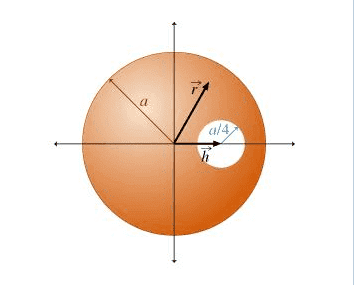# A Charged Sphere with a Cavity

• kbwelch17
In summary, the electric field inside the sphere with no cavity (for r<a) is E = rρ/(3ϵ0), but the electric field inside the sphere with a cavity is zero.

## Homework Statement

An insulating sphere of radius a, centered at the origin, has a uniform volume charge density ρ.

A spherical cavity is excised from the inside of the sphere. The cavity has radius a/4 and is centered at position h(vector) , where |h(vector) |<(3/4)a, so that the entire cavity is contained within the larger sphere. Find the electric field inside the cavity.
Express your answer as a vector in terms of any or all of ρ (Greek letter rho), ϵ0, r(vector) , and h(vector) .

Here is the photo:## Homework Equations

Electric Flux = ∫ E dot dA = Qencl / ϵ0

## The Attempt at a Solution

The first part of the problem asked that we find the electric field inside the sphere with no cavity (for r<a) in terms of the position vector r. I calculated this by using Gauss' Law where dA = 4*pi*r^2 and q = ρ(4/3)r^3*pi. The correct answer was E = rρ/(3ϵ0)

I am running into trouble with this second part. If you set a Gaussian closed surface inside the cavity of the charged insulator, all of the electric field lines that go into it will not terminate inside it. Secondly, no electric field lines originate in the cavity. Therefore, the total charge inside the surface should be 0 and the electric field should be zero. This, however, is not the correct answer (It says it would be if the sphere were a conductor, but it is an insulator). I do not understand why this is so.

The flux can be zero because ##\vec{E}=0##, but just because the flux is 0 doesn't require ##\vec{E}=0##. In this case, all the field lines that enter the volume also exit the volume so the total flux is zero.

Try think of this as a superposition of two spheres of charge, one with charge density ##\rho## and the other with charge density ##-\rho##.

Last edited:
So since charge is distributed throughout the entire sphere, if we performed the same procedure as the first question on the new sphere with a cavity the electric field would be smaller. Since a smaller sphere with the same dimensions of the cavity except with a negative charge density would have a charge E = -ρ(r-h)/(3ϵ0), then the new total sphere charge would be E = (ρr/(3ϵ0))-(ρ(r-h)/(3ϵ0))?

kbwelch17 said:
So since charge is distributed throughout the entire sphere, if we performed the same procedure as the first question on the new sphere with a cavity the electric field would be smaller. Since a smaller sphere with the same dimensions of the cavity except with a negative charge density would have a charge E = -ρ(r-h)/(3ϵ0), then the new total sphere charge would be E = (ρr/(3ϵ0))-(ρ(r-h)/(3ϵ0))?
Right, but ##\vec{E}## is the electric field, not the charge.

•1 person
Okay. I am still struggling to understand why this is different in an insulator and a conductor.

It's simply because in a conductor, you can't have a uniform distribution of charge. As you've probably been taught, all the charge will collect on the surface of a conductor. Why is this the case? What's the difference between an insulator and a conductor?

I don't know if the OP noticed but the final result is interesting. :)